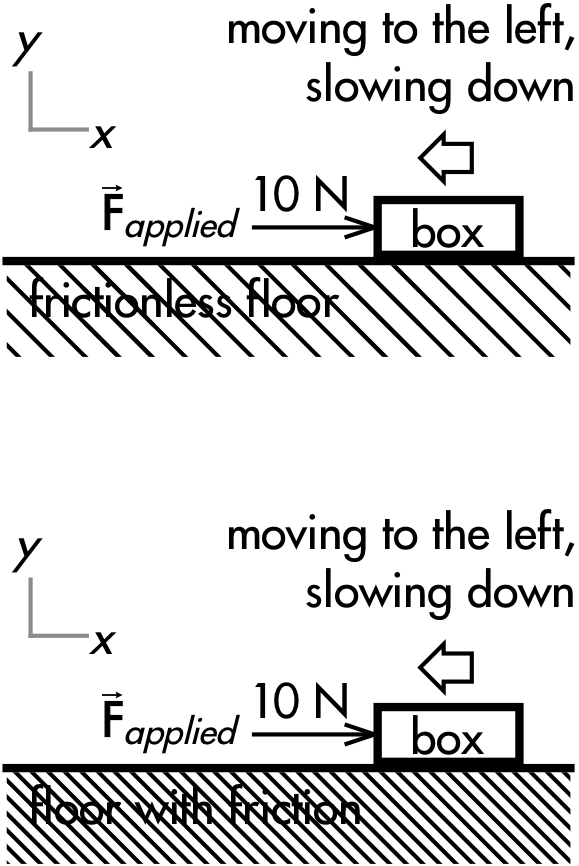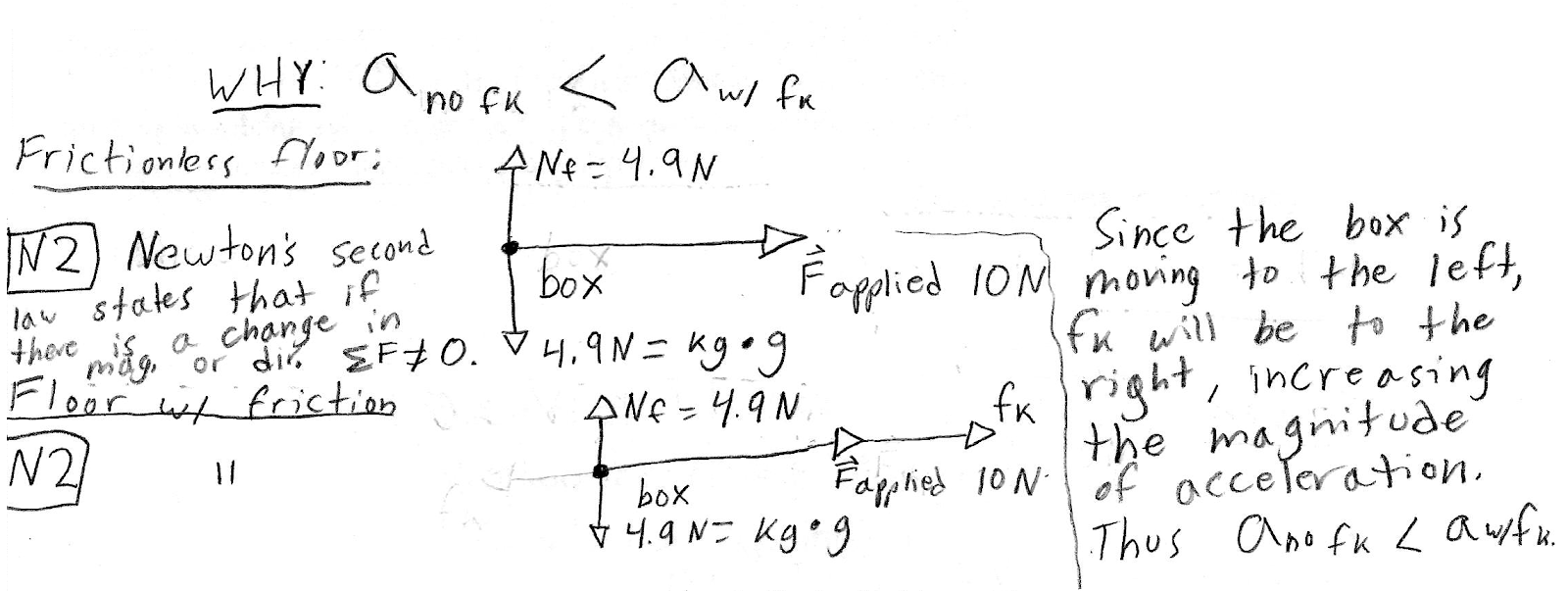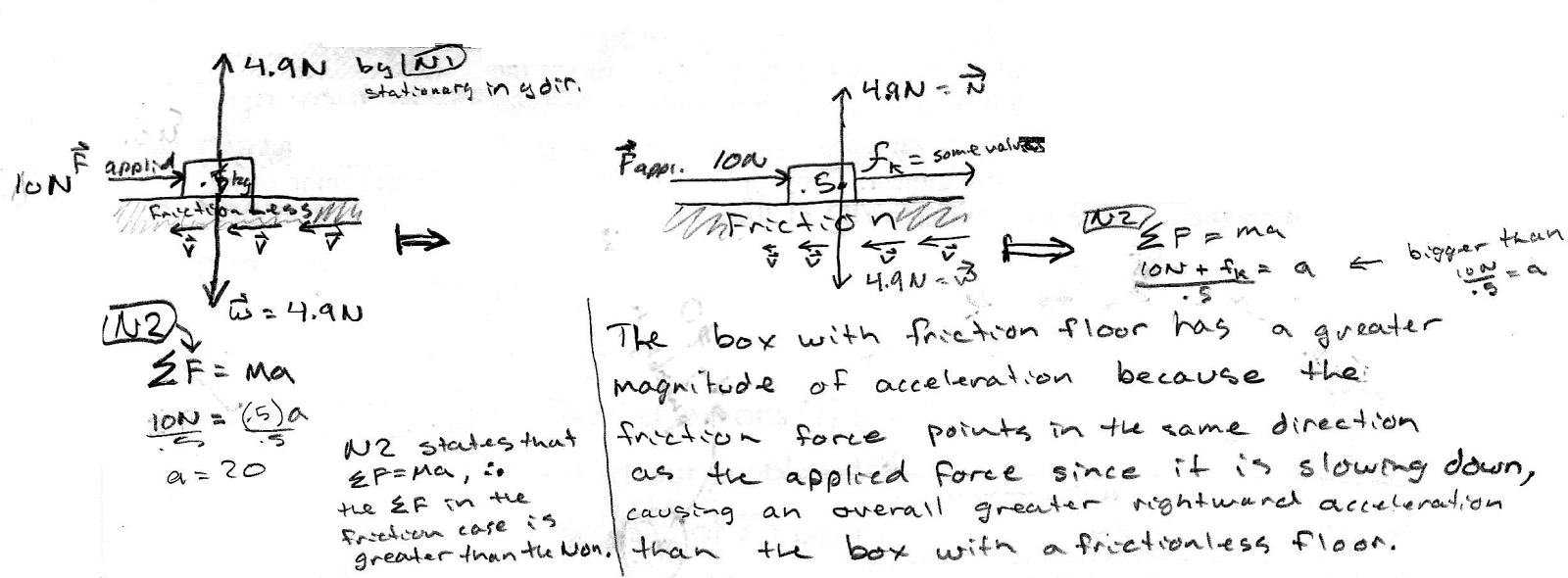## 20171020

### Physics midterm question: comparing accelerations of sliding boxes

Physics 205A Midterm 1, fall semester 2017
Cuesta College, San Luis Obispo, CATwo 0.5 kg boxes are moving to the left, both slowing down while pushed to the right by an applied force of 10 N. Discuss why the box sliding on a frictionless floor will have a smaller magnitude of acceleration than the box sliding on a floor that has friction. Explain your reasoning using free-body diagram(s), the properties of forces, and Newton's laws.

• p:
Correct. Complete free-body diagrams, and discusses/demonstrates:
1. because both boxes are slowing down while moving to the left, Newton's second law applies along the horizontal direction, such that the net force (and acceleration) for each box must point to the right; and
2. for the box on the frictionless floor, there is only horizontal force acting on the box:
applied force Fapplied on the box (10 N, to the right),
such that the net force on this box must be 10 N to the right; and
3. for the box on the floor with friction, there are two horizontal forces acting on the box:
applied force Fapplied on the box (10 N, to the right),
kinetic friction force fk of floor on the box (? N, to the right),
and since these two forces act in the same direction, the net force on this box is the addition of these two force magnitudes (10 N + ? N), and thus must be greater than 10 N;
4. since the boxes have the same mass, the box with the greater net force magnitude will have the greater acceleration magnitude.
(May have only implied (1) and (4) above, and/or ignored discussion of vertical forces and Newton's first law, as long as discussion of (2)-(3) is clear and complete.)
• r:
As (p), but argument indirectly, weakly, or only by definition supports the statement to be proven, or has minor inconsistencies or loopholes. May have kinetic friction (or other) force vectors incorrectly drawn in opposite directions, additional forces (or other types of vectors) added in; but at least recognizes that for the box on the frictionless floor there is only one force that contributes to the net force, while for the box on the floor with friction there are two forces that contribute to the net force.
• t:
Nearly correct, but argument has conceptual errors, or is incomplete.
• v:
Limited relevant discussion of supporting evidence of at least some merit, but in an inconsistent or unclear manner. Some garbled attempt at applying Newton's laws to free-body diagram(s).
• x:
Implementation of ideas, but credit given for effort rather than merit. No systematic application of Newton's laws to the forces on free-body diagram(s).
• y:
Irrelevant discussion/effectively blank.
• z:
Blank.
Sections 70854, 70855
Exam code: midterm01mOoL
p: 14 students
r: 19 students
t: 5 students
v: 14 students
x: 1 student
y: 0 students
z: 0 students

A sample "p" response (from student):Another sample "p" response (from student):Yet nother sample "p" response (from student):